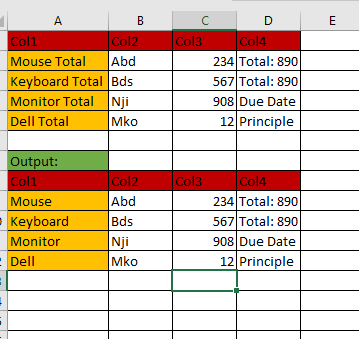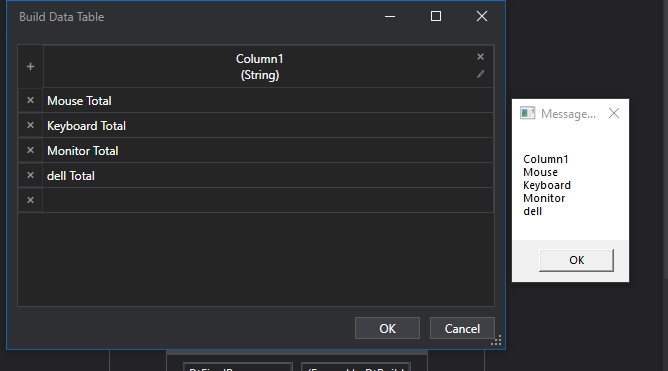# Replace one value with another in a specific columnHi All,

I tried most of expressions

(From r In dtTempFilter.AsEnumerable Select ia = r(0).ToString.trim.Replace(" Total",“”) )
The above one gives an array of strings, I donno how to convert to datatable

(From r In dtFilterTable.AsEnumerable Select ia = r.ItemArray.toList Select ic = ia.ConvertAll(Function (e) e.ToString.Trim.Replace(" Total",“”)).toArray() Select dtResult.Rows.Add(ic)).CopyToDataTable()
The above one changes all ‘Total’ values into “” including last column.

Thanks and Regards,
Sunil M.

we would recommend to loop over the data table e.g

• for each row acitvity - row is the loop variable for the row
• Assign Activity: row(“Col1”) = row(“Col1”).toString.Replace(" Total",“”)

for learning purpose A LINQ within the datatable reconstruction approach would look like:

dtResult = dtData.Clone

dtResult =

``````(From d in dtData.AsEnumerable
let c1 = d(0).toString.Replace(" Total",""))
Let ra = d.ItemArray.Skip(1).Prepend(c1).toArray
``````
1 Like

``````(From d In DtBuild.AsEnumerable()
Let ra = d.ItemArray.Select(Function (x) x.toString.Replace("Total","")).toArray
``````

Regards
Gokul

For learning purpose and explore the different options to update a column value have a look here:

And fo LINQ here:

this statement is replacing in all columns as it is driven by the itemArray and is similar to

``````(From d In DtBuild.AsEnumerable()
Let ra = d.ItemArray.Select(Function (x) x.toString.Replace("Total","")).toArray
``````

As applied here:

Check out the XAML file

Find&Replace.xaml (11.3 KB)

OutputRegards
Gokul

Another Solution Using Studio X activities

Before that Make sure you must enable the Modern activities

Check out the XAML file

Find And Replace.xaml (11.0 KB)Regards
Gokul

Hi @Sunil_Kumar_Marri1
2- Use for each over datatable
3-Use * Assign Activity: row(“Col1”) = Spilt(ow(“Col1”).toString,“ ”)(0)

Thank you so much…)

Thank you so much sir…)

It is worked on first column, but what if that column in middle and one more thing

(From d in dtData.AsEnumerable
Let ra = d.ItemArray.Skip(1).Prepend(c1).toArray

I’m doing this for empty row replace with “Mapping Not Found”,but gets an error.
It would be helpful for my project.

@Sunil_Kumar_Marri1 Then instead of d(0) you can put other column number

Let ra = d.ItemArray.Skip(1).Prepend(c1).toArray

Here, we skip the first column and prepend our manipulated data, I asked this.
``````(From d in dtData.AsEnumerable